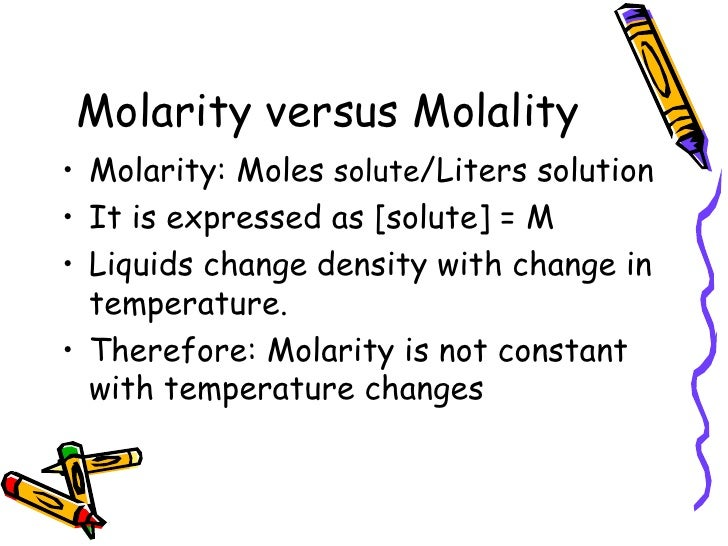# Measuring Concentration

## Key Questions

Molarity changes with temperature.

#### Explanation:

Molarity changes with temperature.

Molarity is moles of solute per litre of solution. Water expands as the temperature increases, so the volume of the solution also increases. You have the same number of moles in more litres, so the molarity is less at higher temperatures.

EXAMPLE

Assume that you have a solution that contains 0.2500 mol of NaOH in 1.000 L of solution (0.2500 M NaOH) at 10 °C. At 30 °C, the volume of the solution is 1.005 L, so the molarity at 30 °C is

$\frac{0.2500 m o l}{1.005 L}$ = 0.2488 M

This may not seem like a big difference, but it is important when you need more than two significant figures in a calculation.

MORAL: If you use molarities in your calculations, make sure that they are all measured at the same temperature.Molarity is the concentration of a solution expressed as the number of moles of solute per litre of solution.

#### Explanation:

To get the molarity, you divide the moles of solute by the litres of solution.

$\text{Molarity" = "moles of solute"/"litres of solution}$

For example, a 0.25 mol/L NaOH solution contains 0.25 mol of sodium hydroxide in every litre of solution.

To calculate the molarity of a solution, you need to know the number of moles of solute and the total volume of the solution.

To calculate molarity:

1. Calculate the number of moles of solute present.
2. Calculate the number of litres of solution present.
3. Divide the number of moles of solute by the number of litres of solution.

EXAMPLE:

What is the molarity of a solution prepared by dissolving 15.0 g of NaOH in enough water to make a total of 225 mL of solution?

Solution:

1 mol of NaOH has a mass of 40.00 g, so

$\text{Moles of NaOH" = 15.0 cancel("g NaOH") × "1 mol NaOH"/(40.00 cancel("g NaOH")) = "0.375 mol NaOH}$

$\text{Litres of solution" = 225 cancel("mL soln") × "1 L soln"/(1000 cancel("mL soln")) = "0.225 L soln}$

$\text{Molarity" = "moles of solute"/"litres of solution" = "0.375 mol"/"0.225 L" = "1.67 mol/L}$

Some students prefer to use a "molarity triangle".It summarizes the molarity formulas as

$\text{Moles" = "molarity × litres}$

$\text{Molarity" = "moles"/"litres}$

$\text{Litres" = "moles"/"molarity}$

• $C = \frac{n}{V}$

C is the concentration
n is the number of moles and
V is the volume of solution

So firstly you need to know what is in the solution, I'll take HCl as an example:

Say you have 5g of HCl in a 500ml solution. You must first find the number of moles which is the mass in grams divide by the molar mass of HCl which would be 36.45g/mole. (1g/mole is Hydrogen and 35.45g/mole is Cl)

You get that answer then you divide by your volume in litres, which is 0.5L.

Your answer's unit will mol/L which is sometimes also known as just M.

Should get an answer of 0.27 mol/L for this example :)

Hope I helped :)